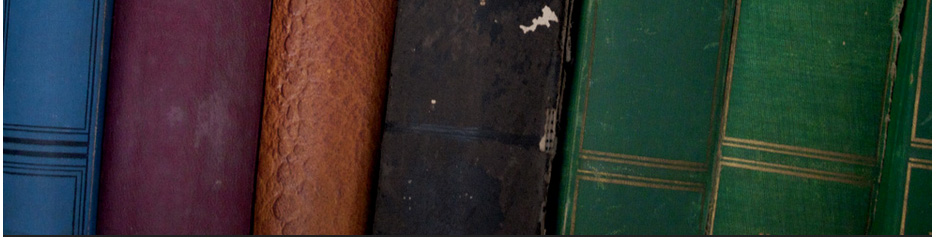## Secondary educationks3 Maths video collections

Algebra

ks30001 Expressions, Equations, Formulae & Identities

ks30002 Forming algebraic expressions

ks30003  KEY STAGE 3 -- ALGEBRA/

ks30005 Straight line graphs 1

ks30006 Coordinates and shapes - Corbettmaths

ks30007 System of Linear Inequalities

ks30008  Graphing Systems of Linear Inequalities

ks30010 Solving Simultaneous Equations

ks30011 Solving quadratic equations

ks30012 Solving quadratics by factoring

ks30013  How to Solve Quadratic Equations

ks30015 Completing the square

Statistics and probability

ks30001 Statistics - Probability Intro

ks30002 Probability & Statistics (1 of 62) Introduction

ks30008  Probability and Statistics

Ermias Home

HAREP The Horn of Africa Research and Knowledge Exchange Platform © 2010-2012

 Contact: rkidane@talk21.com Please click Here to send your feedback